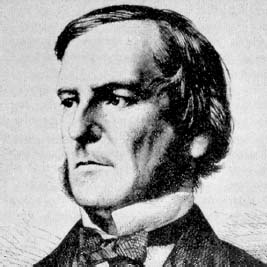NextPrevious

# Who was Karl Friedrich Gauss?

German mathematician, physicist, and astronomer Karl Friedrich Gauss (1777-1855; also seen as Johann Carl [or Karl] Friedrich Gauss) was considered one of the greatest mathematicians of his time—some have even compared him to Archimedes and Newton. His greatest mathematical contributions were in the fields of higher arithmetic and number theory. He discovered the law of quadratic reciprocity; determined the method of least squares (independently of French mathematician Adrien-Marie Legendre [1752-1833]); popularized the symbol “i” as the square root of negative 1 (although Euler first used the symbol); did extensive investigations in the theory of space curves and surfaces; made contributions to differential geometry; and the list goes on. In 1801, after the discovery (and subsequent loss) of the first asteroid, Ceres, by Giuseppe Piazzi, Gauss calculated the object’s orbit with little data; the asteroid was found again thanks to his calculations. He further calculated the orbits of asteroids found over the next few years.English mathematician George Boole was the first to develop ideas on symbolic logic, that is, the use of symbols to represent logical principles.

Close

This is a web preview of the "The Handy Math Answer Book" app. Many features only work on your mobile device. If you like what you see, we hope you will consider buying. Get the App